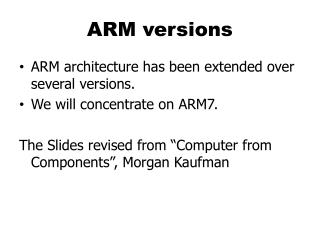DownloadDownload PresentationARM versions

# ARM versions

Download Presentation## ARM versions

- - - - - - - - - - - - - - - - - - - - - - - - - - - E N D - - - - - - - - - - - - - - - - - - - - - - - - - - -
##### Presentation Transcript

1. ARM versions • ARM architecture has been extended over several versions. • We will concentrate on ARM7. The Slides revised from “Computer from Components”, Morgan Kaufman

2. ARM ISA • All instructions are 32-bit long (not include Thumb) • Register and memory word are 32-bit • Memory address is 32-bit • Byte addressed • Can be configured at power-up as either little- or bit-endian mode Note: There is a 64-bit ARM ISA Overheads for Computers as Components

3. N Z C V ARM Registers r0 r8 r1 r9 0 31 r2 r10 CPSR r3 r11 r4 r12 r5 r13 (SP) r6 r14 (LR) r7 r15 (PC) CPSR: Current Program Status Register

4. Status bits • Every arithmetic, logical, or shifting operation sets CPSR bits: • N (negative), Z (zero), C (carry), V (overflow). • Examples: • -1 + 1 = 0: NZCV = 0110. • 231-1+1 = -231: NZCV = 1001.

5. ARM data instructions • Basic format: ADD r0,r1,r2 • Computes r1+r2, stores in r0. • Immediate operand: ADD r0,r1,#2 • Computes r1+2, stores in r0.

6. ARM data instructions • ADD, ADC : add (w. carry) • SUB, SBC : subtract (w. carry) • RSB, RSC : reverse subtract (w. carry) • MUL, MLA : multiply (and accumulate) • AND, ORR, EOR • BIC : bit clear • LSL, LSR : logical shift left/right • ASL, ASR : arithmetic shift left/right • ROR : rotate right • RRX : rotate right extended with C Overheads for Computers as Components

7. Load/store instructions • LDR, LDRH, LDRB : load (half-word, byte) • STR, STRH, STRB : store (half-word, byte)

8. Address modes • Register indirect : LDR r0,[r1] • With two registers : LDR r0,[r1,r2] • With 2nd register negated: LDR r0,[r1,-r2] • With 2nd register shifted: LDR r0,[r1,-r2,LSL#2] • with a base register and 12-bit offset: LDR r0,[r1,#4]

9. Address modes • Register indirect : LDR r0,[r1] • With two registers : LDR r0,[r1,r2] • With 2nd register negated: LDR r0,[r1,-r2] • With 2nd register shifted: LDR r0,[r1,-r2,LSL#2] • with a base register and 12-bit offset: LDR r0,[r1,#4]

10. LDR and STR as pseudo-instructions • LDR and STR may also be pseudo-instructions LDR r1, label STD r1, label • R15/PC is used as the based register, and label is encode as its offset to PC • They work if and only if label is within [-4095, +4095] of PC

11. Array Access • Assume X in r0, i in r1, a in r2, all int type a = X; LDR r2, [r1] a = X; LDR r2, [r1,#8] a = X[i]; LDR r2, [r0,r1,LSL #2] LSL: Logic shift left

12. Example: C assignments • C: c = a + b; • Assembler: LDR r0, a ; get value of a LDR r1, b ; get value of b ADD r3,r0,r1 ; compute a+b STR r3, c ; c = a+b

13. ARM Condition and Branch • All instructions can be conditionally executed, testing CPSR: • EQ, NE, CS, CC, MI, PL, VS, VC, HI, LS, GE, LT, GT, LE • Example, execute if EQ: ADDEQ r1, r2, r3 • Branch operation: B #100 • Can be performed conditionally • Example: BEQ Branch if equal • BEQ is the B instruction with condition code EQ

14. Example: if statement if (a > b) { c = a; } else { c = b; } ; compute and test condition LDR r0, a ; get value of a LDR r1, b ; get value for b CMP r0,r1 ; compare a < b BLE fblock ; if a <= b, branch to else STR r0, c ; c = a B endif else: STR r1, c ; c = b endif:

15. Conditional Instruction Implementation if (a > b) { c = a; } else { c = b; } ; compute and test condition LDR r0, a ; get value of a LDR r1, b ; get value for b CMP r0,r1 ; compare a < b STRGTr0, c ; if GT, c = a STRLEr1, c ; if LE, c = b

16. ARM subroutine linkage • Branch and link instruction: BL foo • Copies current PC to r14. • Equivalent to MIPS jal • To return from subroutine: MOV r15,r14 • r15 is the PC in MIPS

17. ARM Call Convention In MIPS terms • r0 – r3: Parameters and return value, also temporary • r4 – r8, r10, r11: Saved temporary • r9: Usage is Platform-dependent • r12: Temporary • r13: Stack pointer, preserved • r14: Link register • r15: The program counter

18. Extra Slides